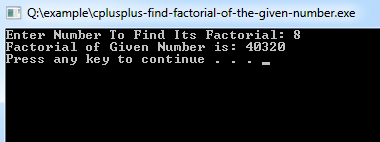## C++ Programming Examples Tutorial Index

This C++ program is used to demonstrates calculate the factorial of any given number input by the user.

Example:

``````#include <iostream>
using namespace std;

int main()
{
int number,factorial=1;
cout << "Enter Number To Find Its Factorial: "; cin>>number;

for(int i=1;i<=number;i++)
{
factorial=factorial*i;
}

cout<<"Factorial of Given Number is: " << factorial << endl;
system("PAUSE");
return 0;
}``````

Program Output:Explanation:

First of all, you have to include the iostream header file using the "include" preceding by # which tells that hat the header file needs to be process before compilation, hence named preprocessor directive. Now, for removing naming conflict you can use namespace statement within a program.

Then the main() function is declared with return type as integer. Now you have to take two integer variables name number, factorial and initialize the value of factorial as 1. Then the cout<<""; statement is used to print the following message / string:
"Enter Number To Find Its Factorial:"

In consecutive, cin>> statement is used to fetch the input from the user (mainly using a keyboard). Now a for loop is introduced which makes the iteration possible having value i=1 to the choose number of the user. Inside the for loop, the value of 'factorial' is initialized by multiplying the variable 'factorial' with 'i'.

Finally the value of 'factorial' gets displayed using cout statement as a form of output. The system("PAUSE") is used to pause the console of the system. And finally the return 0; statement is used to return an integer type value back to main().